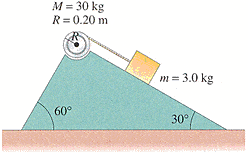# Block sliding down an incline plane with a cord connected to a cylinder.

• unrulypanda

## Homework Statement

A cord connected at one end to a block which can slide on an inclined plane has its other end wrapped around a cylinder resting in a depression at the top of the plane as shown in the figure. Determine the speed of the block after it has traveled 1.70 m along the plane, starting from rest for the following circumstances.(a) Assume there is no friction.
(b) Assume the coefficient of friction between all surfaces is μ = 0.035. [Hint: First determine the normal force on the cylinder, and make any reasonable assumptions needed.]

## The Attempt at a Solution

Where should I start? I know this has something to do with torque but I don't know how to calculate it.